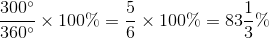# Intermediate Geometry : How to find the percentage of a sector from an angle

## Example Questions

2 Next →

### Example Question #11 : How To Find The Percentage Of A Sector From An Angle

A survey was given to 1,000 middle school students across the United States, asking them to choose their favorite subject in school.  The results of the survey are below, with each subject followed by the number of degrees composing that subject's sector in the circle graph displaying this data.

Math, 75 degrees

English, 58 degrees

Science, 62 degrees

Social Studies, 100 degrees

Music, Art or PE: 65 degrees

What percent of students prefer a subject that is not Music, Art or PE?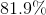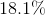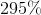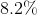Explanation:

First, add together the degrees of the sectors representing Math, English, Science and Social Studies.

Take this sum, 295 and set-up a proportion: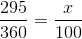and solve for.

### Example Question #12 : How To Find The Percentage Of A Sector From An Angle

Reference a map of major cities on the West Coast to help you with this question!

A survey was given to fans throughout the West Coast, asking them who their favorite team was.  The results are below with the team name and the degrees making up the sector representing that team in a circle graph that displayed this data.

Team A: 123 degrees

Team B: 89 degrees

Team C: 36 degrees

Team D: 20 degrees

Other: 92 degrees

What percent of fans prefere Team A, C, or D?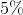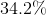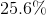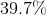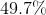Explanation:

First add together the degrees from the sectors representing Teams A, C, and D.

Take this sum, 179 and set-up a proportion: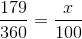and solve for.

### Example Question #13 : How To Find The Percentage Of A Sector From An Angle

A pie chart depicts the favorite beverages of high schoolers.  Below is the data with the beverage and the number of degrees making up that beverage's sector.

Soda: 86 degrees

Diet Soda: 44 degrees

Water: 55 degrees

Juice: 99 degrees

Other: ? degrees

What percent of high schoolers prefer a beverage that was not specifically listed as a choice?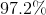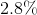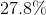Explanation:

First add together the known sectors.  Use that sum (350 degrees) and subtract from the total number of degrees in a circle (360 degrees).

Take that difference and set-up a proportion: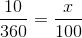and solve for.

### Example Question #14 : How To Find The Percentage Of A Sector From An Angle

A circle graph displays data of the eye colors of a group of middle schoolers.  The data is shown below with the eye color followed by the number of degrees composing that sector.

Brown: 208 degrees

Green: 20 degrees

Blue: 25 degrees

Hazel: 50 degrees

Other: 57 degrees

What percent of students have brown eyes?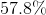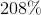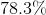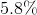Explanation:

Take the degrees representing the brown eye sector and set-up a proportion: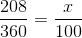and solve for.

### Example Question #15 : How To Find The Percentage Of A Sector From An Angle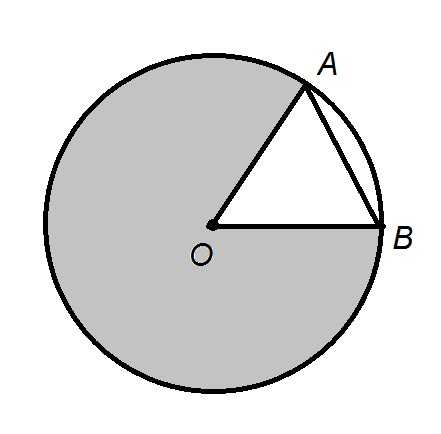In the above diagram,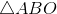is an equilateral triangle. What percent of the circle has been shaded in?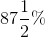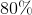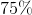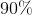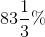The three interior angles of equilateral triangle- in particular,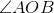- measure. This makes the unshaded sector of the circle a sector with acentral angle, or, equivalently, asector. The shaded sector is therefore one of measure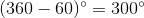. It is therefore